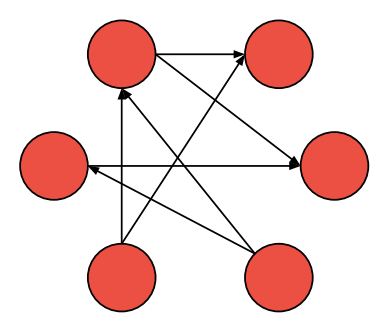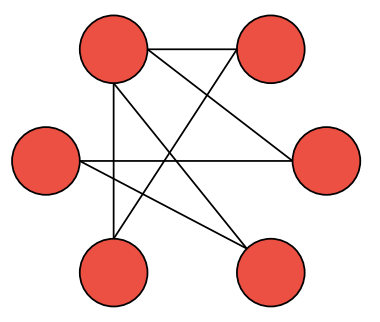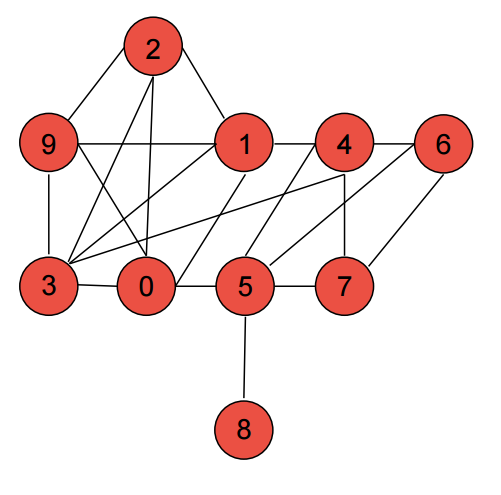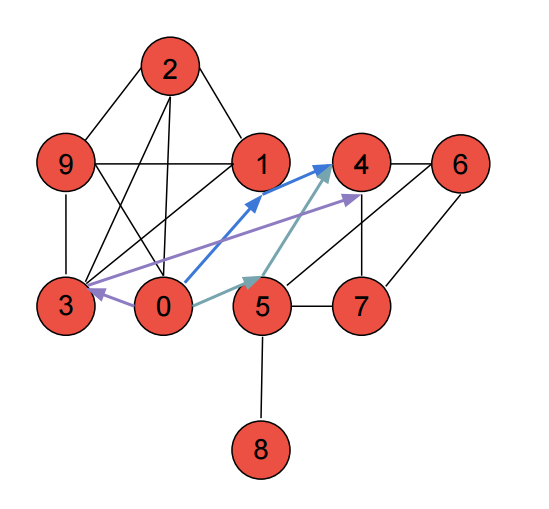Welcome to a brand new blog series! Each post will consist of a computer science algorithm and a real world application. Additionally, if you are interested in machine learning and have not yet seen the previous series, go check it out!

Todays topic will focus on graphs. To apply this knowledge, we will construct a social network by utilizing an undirected graph and provide friend recommendations through mutual friendship. As you probably know, the mutual friends feature is engrained in Facebook.

# Graphs

One popular example of a graph is the internet.

In computer science terms, a graph is a network of nodes that are connected together. These “connections” between nodes are called edges. One popular example of a graph is the internet: each node is a host (personal computer or server), and the edges are the pathways that connect these hosts together.

Graphs can be classified into two different categories: directed and undirected. A directed graph is composed of a unidirectional path between two nodes. For graph G where G contains nodes A and B, if A is connected to B, A can travel to B, but B cannot travel to A. Simply put, a directed graph is like a one-way street – traffic can only flow in one direction.An example of a directed graph.

Likewise, an undirected graph is comprised of a bidirectional path between two nodes. This means that for graph G, A can travel to B and B can travel to A. In short, an undirected graph is like a two-way street. This type of graph can be used to represent Facebook friendships: not only are you friends with someone else, but they are also friends with you.An example of an undirected graph.

# Mutual Friends

If you own a Facebook account, you have probably noticed the mutual friend count below each friend recommendation. When Facebook offers friend suggestions, you will most likely be presented friends in decreasing order by number of mutual friends. We will recreate the mutual friendship counter while attempting to solve this problem with optimal asymptotic complexity. The full source can be found on Github.

Let’s take a simple social network of 10 people.The network of 10 people that we will be working with.

The premise of our first file, Network.java, is to construct a list of neighbors for each node. Each edge represents two indices in the neighbor list (connecting A to B and B to A).

We utilize an adjacency matrix as well as an adjacency list. Adjacency lists allow us to iterate through each set of neighbors efficiently, while the adjacency matrix allows us to determine whether one person is friends with another in constant time. Depending on how you interact with graph relationships, be sure to consider the pros and cons between adjacency lists and matrices.

Once we have created the network of people, we can start processing the mutual friendships for each node.

The concept is to first iterate over each node and add each friend (of the current node) to a queue. After, we will process each element with a TreeMap to determine the mutual friend nodes. We use a TreeMap so that we can populate a list of mutual friends in descending order without having to sort afterwards. TreeMap uses a Red-Black tree as the underlying algorithm – thus we get O(log(n)) for insertion. When we multiply the insertion cost by the cost of the two node loops, we get an upper bound of O(n^2*log(n)).

The worst case is when a complete graph is used. A complete graph contains the largest possible number of edges – thus each node is connected to every other node. The number of edges in a complete graph is equal to n(n-1)/2 where n is the amount of nodes.

To check this algorithm, let’s compare the output of the application with the graph below. We will compare one of the results together. Confirming the rest will be an exercise for you ?. Nodes 0 and 4 tie for the largest amount of mutual friends, therefore we will analyze this case. With the graph illustration below, we see that there should be 3 mutual friends.

``````0 {4=3, 6=1, 7=1, 8=1}
1 {5=2, 6=1, 7=1}
2 {4=2, 5=1}
3 {5=2, 6=1, 7=1}
4 {0=3, 2=2, 8=1, 9=2}
5 {1=2, 2=1, 3=2, 9=1}
6 {0=1, 1=1, 3=1, 8=1}
7 {0=1, 1=1, 3=1, 8=1}
8 {0=1, 4=1, 6=1, 7=1}
9 {4=2, 5=1}
``````

In the first line of the output (node 0), we see that node 4 shares 3 mutual friends. Likewise, we can note that the fifth line (node 4) contains node 0 and observing that node 0 also contains 3 mutual friends. Finally, by looking at the illustration below, we can confirm the results.Illustrating paths for mutual friendship. 0 and 4 have three mutual friends.

# Conclusion

Graphs are a summed up as a network of nodes which are connected by edges. Furthermore, each graph can be classified either as a directed graph, or an undirected graph. Directed graphs are like a one-way street, while a directed graph is like a two-way street. Our mutual friend implementation uses a directed graph since the friend-friend relationship is a “two-way street”. Finally, the worst case of this algorithm is when a complete graph is exercised.

I hope you enjoyed this article, and be sure to check in next time!